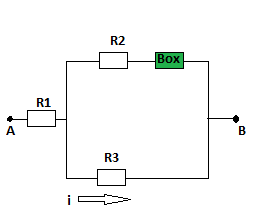The figure shows a section of a circuit. The resistances are R_1 = 2.6 \space \Omega, R_2 = 4.6...

Question:

The figure shows a section of a circuit. The resistances are {eq}R_1 = 2.6 \space \Omega, R_2 = 4.6 \space \Omega,, R_3 = 6.1 \space \Omega, {/eq} and the indicated current is {eq}i = 6.5 \space A {/eq}. The electric potential difference between points A and B that connect the section to the rest of the circuit is {eq}V_A - V_B = 79 \space V {/eq}.

a. Is the device represented by "Box" absorbing or providing energy to the circuit and

b. at what rate?Kirchoff's Current Law:

Kirchoff's Current Law provides us with a useful way to track the currents as they branch in a circuit. According to the law, the current entering a junction or a node is equal to the sum of the currents leaving that junction or node.

Become a Study.com member to unlock this answer!

Part (a)

The potential difference V across the parallel section of the circuit is the product of the current i and resistance {eq}R_3 {/eq} in...Parallel Circuits: Calculating Currents with Ohm's Law

from

Chapter 9 / Lesson 6
2.6K

Understand Kirchhoff's law and Ohm's law for parallel circuits, and see how to find current in a parallel circuit using the parallel circuit current formula.